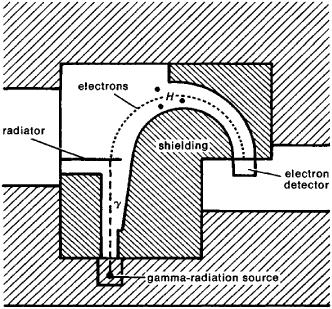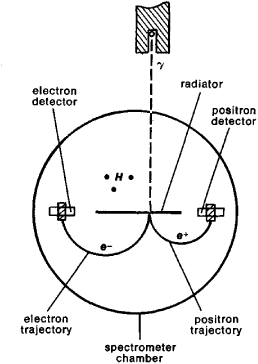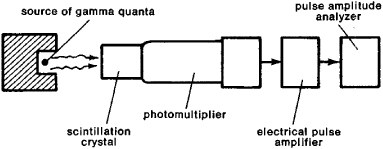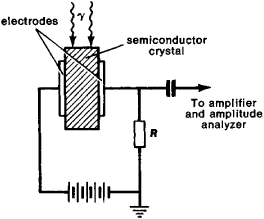# Gamma Spectrometer

## Gamma Spectrometer

an instrument for measuring gamma-radiation spectra. In most gamma spectrometers the energy and intensity of a stream of gamma quanta are not determined directly but by measuring the energy and intensity of a stream of secondary charged particles that are produced by the interaction of the radiation with a substance. An exception is the crystal-diffraction gamma spectrometer, which measures the wavelength of gamma rays directly.

The main characteristics of gamma spectrometers are their efficiency and resolving power. The efficiency is determined by the probability of formation of a secondary particle and the probability of recording it. The resolving power characterizes the possibility of separating two gamma lines having approximately the same energy. The relative width of a line, which is obtained when measuring monochromatic gamma rays, is usually taken as a measure of the resolving power; it is determined quantitatively by the ratio Δ&& where & is the energy of the secondary particles and Δ& is the line width at half its height (in energy units).

In magnetic gamma spectrometers the secondary particles are produced upon the absorption of gamma quanta in a so-called radiator; their energy is measured in the same way as in the magnetic beta spectrometer (see Figure 1).Figure 1. Diagram of a magnetic gamma spectrometer. In a magnetic field H perpendicular to the plane of the figure, secondary electrons move in circular paths whose radii are determined by the electron energies and the magnetic field H. When the field changes, the detector records electrons of various energies. The crosshatching indicates lead shielding.

The size of the magnetic field H in the spectrometer and the radius of curvature ρ of the electrons’ trajectory determine the energy & of the electrons recorded by the detector. If the radiator is made out of a substance having a low atomic number, the secondary electrons are formed mainly as a result of the Compton effect; if the radiator is made from a heavy substance (lead or uranium) and the energy of the gamma quanta is low, the secondary electrons will then be produced mainly by the photoelectric effect. At energies hv ≥ 1.02 mega electron volts (MeV), the production of electron-positron pairs by the gamma quanta becomes possible. A magnetic pair gamma spectrometer is shown in Figure 2. The pairs are produced in a thin radiator located in a vacuum chamber. The measurement of the sum of the electron and positron energies makes possible the determination of the energy of a gamma quantum. Magnetic gamma spectrometers have a high resolving power (usually in the order of 1 percent or fractions of a percent), but their efficiency is low, so that it is necessary to use highly active gamma-radiation sources.Figure 2. Diagram of a pair gamma spectrometer. In a homogeneous magnetic field H perpendicular to the plane of the figure, electrons and positrons move along circular paths in opposite directions.

In scintillation gamma spectrometers the secondary electrons are produced upon the interaction of gamma quanta with a scintillator (a substance in which the secondary electrons excite fluorescence). The light flash is converted into an electrical impulse by a photomultiplier (see Figure 3); the magnitude of the signal produced by the multiplier is proportional to the energy of an electron and therefore associated with the energy of the gamma quantum. The amplitude distribution of the signals is measured with special electronic devices (amplitude analyzers).Figure 3. Diagram of a scintillation gamma spectrometer

The efficiency of a scintillation gamma spectrometer depends on the size of the scintillator; it can approach 100 percent at fairly low energies. However, its resolving power is poor. For gamma quanta with an energy of 662 keV, Δ&/& 6 percent, decreasing approximately as &-1/2 with increasing energy &.

The operation of semiconductor gamma spectrometers is based on the production by gamma radiation of electron-hole pairs within a semiconductor crystal (usually germanium with an admixture of lithium). The charge thus produced is collected at electrodes and is recorded as an electrical signal with a value determined by the energy of the gamma quanta (see Figure 4). Such spectrometers have very high resolving power because of the small amount of energy expended in the production of an electron-hole pair. For hv = 662 keV, Δ&/& ~ 0.5 percent. Their efficiency is usually lower than that of scintillation gamma spectrometers, since gamma rays are not absorbed as well in germanium as, for example, in an Nal scintillation crystal. In addition, the size of the semiconductor detectors presently being used is small. Among their drawbacks is also the necessity of cooling them to temperatures close to that of liquid nitrogen.

The highest accuracy of measurement of gamma-quanta energy is provided by the crystal-diffraction gamma spectrometers, in which the wavelength of the gamma radiation is measured directly. Such a gamma spectrometer is similar to the instruments for observation of X-ray diffraction. In passing through quartz or calcite crystals, the radiation is reflected from the crystal planes at various angles, depending on its wavelength, and is recorded by a photographic emulsion or a photon counter. The disadvantage of these instruments is their low efficiency.Figure 4. Diagram of a semiconductor gamma spectrometer

Proportional counters, whose resolving power in the low-energy region is substantially higher than that of scintillation gamma spectrometers, are often used for spectral measurements of low-energy gamma radiation (to 100 keV). When hv > 100 keV, proportional counters are not used, because their efficiency is too low. Gamma-radiation spectra of very high energy are measured with shower detectors, which determine the total energy of the particles in electron-positron showers caused by high-energy gamma quanta. The showers are usually produced in radiators of very large size, which ensure the complete absorption of all secondary particles. The flashes of fluorescence (or Cherenkov radiation) are recorded by a photomultiplier.

In some cases the process of photodisintegration of a deuteron is used to measure the energy of gamma quanta. If that energy exceeds the deuteron’s binding energy (~ 2.23 MeV), the deuteron can be split into a proton and a neutron. The energy of the incident gamma quanta can be determined by measuring the kinetic energy of these particles.

### REFERENCES

Al’fa-, beta- i gamma-spektroskopiia, issue 1. Edited by K. Siegbahn. Moscow, 1969. (Translated from English.)
Metody izmereniia osnovnykh velichin iadernoi fiziki. Moscow, 1964. (Translated from English.)
Kalashnikova, V. I., and M. S. Kozodaev. Detektory elementarnykh chastits. Moscow, 1966. (Eksperimental’nye metody iadernoi fiziki, part 1.)

V. P. PARFENOVA and N. N. DELIAGIN

References in periodicals archive ?
Tenders invited for Supply,installation, testing, demonstration and calibration of gamma spectrometer based on nai (ti) detector with multi channel analyzer(mca) and associated electronics along with latest available data acquisition facility for spectrum processing.
Purpose: Gamma spectrometer systems are complex instruments, and the quality of the numerical results they provide strongly depends on their reliable calibration.
Sample position Gamma spectrometer Result Unit Karshenasan Soil radio nucleotide 877 [+ or -] 33 Bq / kg Kosar Soil radio nucleotide 812 [+ or -] 30 Bq / kg Niar1 Soil radio nucleotide 984 [+ or -] 37 Bq / kg Niar2 Soil radio nucleotide 857 [+ or -] 32 Bq / kg Dash Kasan Soil radio nucleotide 760 [+ or -] 28 Bq / kg Shorabil1 Soil radio nucleotide 638 [+ or -] 24 Bq / kg Shorabil2 Soil radio nucleotide 634 [+ or -] 24 Bq / kg
The area was scanned with the help of radiation monitors called identiFinder gamma spectrometer and RadAlert gamma dosimeter.
The new scintillating bolometer has performed excellently, proving its viability as a detector in experiments to look for dark matter, and also as a gamma spectrometer (a device that measures this type of radiation) to monitor background radiation in these experiments", said Abancens.
Lot No: 1 Part 1 - Gamma spectrometer (including detector shield) for the HPNC converter
For determining activity degree of elements such as U-238, Ra-226, Th-232, Cs-137, and K-40, we sent 4 soil samples to Iran's atomic energy agency laboratory (Jaber-ebn-Hayyan laboratory), and measured by HPGe Gamma spectrometer with germanium detector.
Tenders invited for Supply of Gamma spectrometer based on 3~x3~ nai detector
Tenders are invited for Purchase Of Gamma Spectrometer
Tenders invited for Supply of Portable gamma spectrometer
Tenders are invited for A low-background high-resolution semiconductor gamma spectrometer with a detector of high-purity germanium (202/17 RCSE and OZ repeated)

Site: Follow: Share:
Open / Close